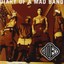MathematicsOpenStudy (anonymous):

WHAT IS THE PRODUCT OF 3.63 AND 6.8 ROUND THE ANSWER TO THE NEAREST CENTOpenStudy (shadowfiend):

So first off, do the multiplication as if there were no decimal points. What's the result?OpenStudy (anonymous):

24684OpenStudy (shadowfiend):

Ok, excellent.OpenStudy (shadowfiend):

Next, move the decimal point to the left one for each place that it was moved to the left in the first number. So 3.63 has the decimal point two places to the left. Then, you do the same thing with the second number. So 6.8 has it one place to the left, so you move the decimal point over a total of three places. What do you have then?OpenStudy (anonymous):

24.684OpenStudy (shadowfiend):

Exactly! Now the last step is to round. Which number is in the cent place?OpenStudy (anonymous):

684 DO I ROUND IT TO 70OpenStudy (shadowfiend):

Not quite. The number in the cents place is 8, specifically. Rounding to the nearest cent means you round the number in the cent spot based on what is to its right. Since 8 is in the cent spot, and 4 is to its right, what do you round the 8 to?OpenStudy (anonymous):

SO IT WOULD BE 680 BCUZZ ITS A 4 NOT A 5 IF IT WAS 5 THEN IT WOULD BE 90 RITEOpenStudy (anonymous):

SO IT WOULD BE 680 BCUZZ ITS A 4 NOT A 5 IF IT WAS 5 THEN IT WOULD BE 90 RITEOpenStudy (shadowfiend):

Exactly.OpenStudy (shadowfiend):

So the result is 24.68.OpenStudy (anonymous):

OOO OK THANKS SOOO MUCH YOUR A BIG HELPOpenStudy (shadowfiend):

Latest QuestionsJoe348: Is it rational to replace Columbus Day with Indigenous Peoples Day?
19 seconds ago 5 Replies 0 MedalsJamesTDG: If f(x) = x^2 u2013 26 and g(x) = x u2013 4, what is the domain of (StartFraction f Over g EndFraction) (x)? Show your work! I changed the equation so I can
10 minutes ago 0 Replies 0 MedalsSprinkles285: Answer the questions below. Use the rubric in the materials for help if needed. Your response should consist of three complete sentences in Spanish.
26 minutes ago 0 Replies 0 Medalskward64: ok i sung i forrogt the name of the song aready but i sung someones song and here it is https://voca.
5 minutes ago 24 Replies 0 MedalsKyledaGreat: Your backpacker's guide contains a grid map of Paris, with each unit on the grid representing 0.
40 minutes ago 4 Replies 0 MedalsTETSXPREME: help
37 minutes ago 2 Replies 0 MedalsNoodlearms: The dashed triangle is the image of the solid triangle. The center of dilation is (6, 6).
27 minutes ago 14 Replies 2 MedalsSprinkles285: When a verb ends in -ar, -er, or -ir, you say that the verb is in the _____ form. future tense conjugated past tense infinitive
1 hour ago 3 Replies 1 MedalNoodlearms: Solve for x.
1 hour ago 14 Replies 0 Medals